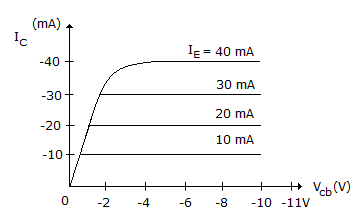# Electronics and Communication Engineering - Electronic Devices and Circuits

6.
Resistivity of metals is expressed in terms of
μ ohm
μ ohm/cm
μ ohm-cm
μ ohm-cm/°C
Explanation:
No answer description is available. Let's discuss.

7.
Figure shows characteristics curves for bipolar transistor. These curves areoutput characteristics of n-p-n transistor (common base)
output characteristics of p-n-p transistor (common base)
output characteristics of n-p-n transistor (common emitter)
output characteristics of p-n-p transistor (common emitter)
Explanation:
No answer description is available. Let's discuss.

8.
Zener diode is invariably used with
forward bias
reverse bias
either (a) or (b)
zero bias
Explanation:
No answer description is available. Let's discuss.

9.
The relation between plate current and plate voltage of a vacuum diode is called
Richardson Dushman equation
Langmuir Child law
Ohm's law
Boltzmann's law